ET大脑

API与工具

# TensorFlow实现图像分类

## 一、背景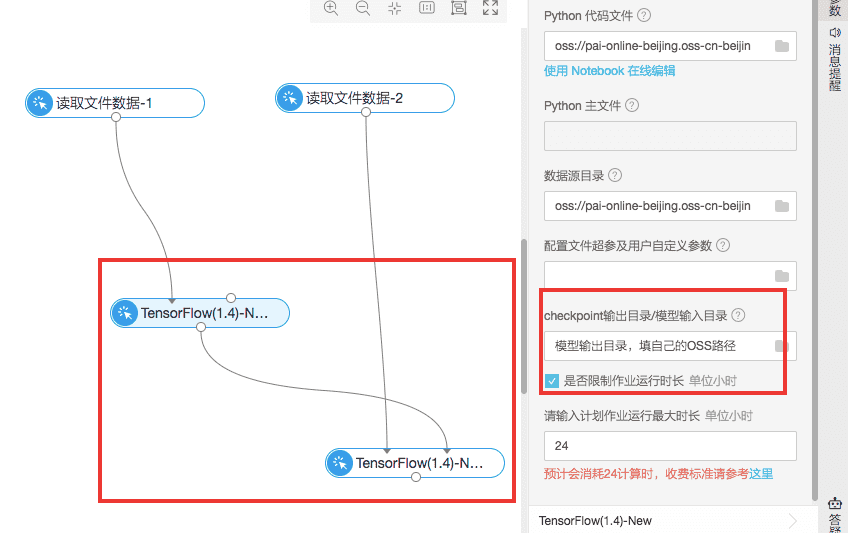## 三、数据探索流程

### 1.数据源准备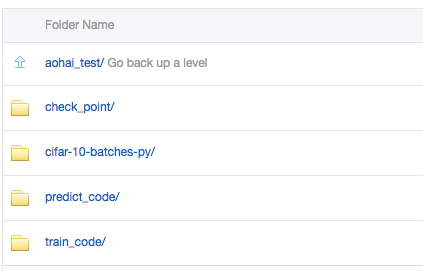• check_point:用来存放实验生成的模型

• cifar-10-batches-py：用来存放训练数据以及预测集数据，对应的是下载下来的数据源cifar-10-batcher-py文件和预测集bird_mount_bluebird.jpg文件

• train_code:用来存放训练数据，也就是cifar_pai.py
• predict_code:用来存放cifar_predict_pai.py

### 3.搭建训练逻辑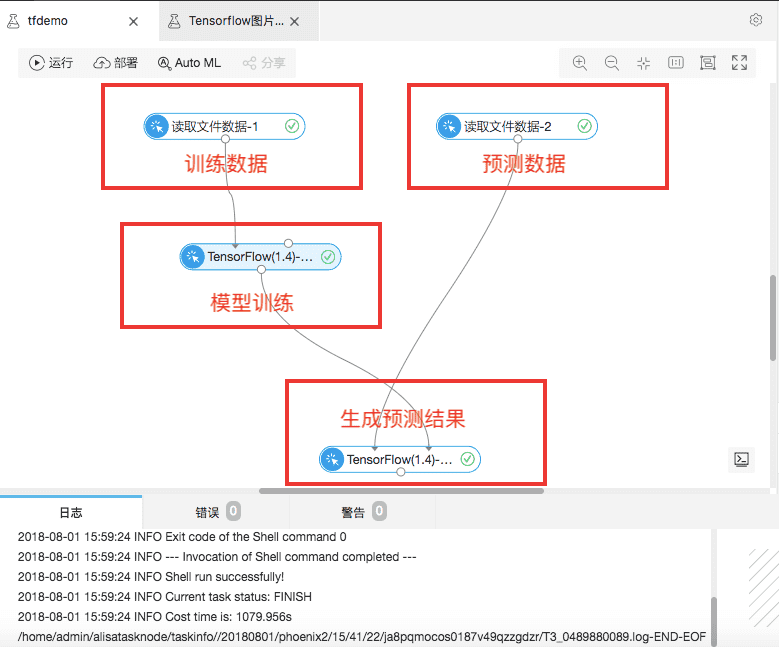### 4.训练/预测数据配置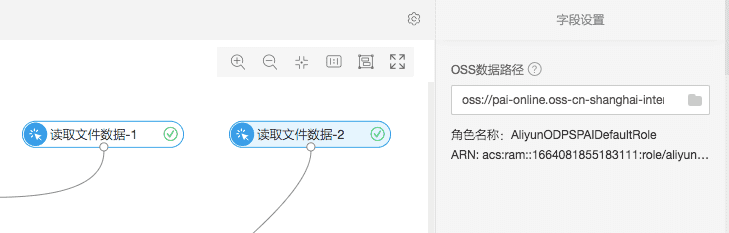### 4.模型训练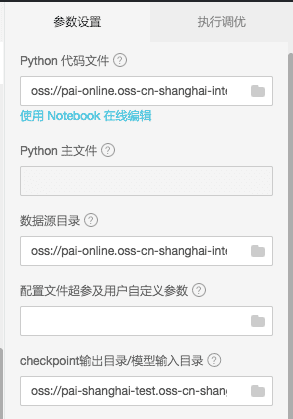• Python代码文件：OSS中的cifar_pai.py
• 数据源目录：OSS中的cifar-10-batches-py文件夹，会自动从上游的“读文件数据“节点同步
• checkpoint输出目录/模型输入目录：OSS中的check_point文件夹，用来输出模型
• 执行调优：用来配置多机多卡相关数据

#### 4.1代码解析

``network = input_data(shape=[None, 32, 32, 3],``                         data_preprocessing=img_prep,``                         data_augmentation=img_aug)``    network = conv_2d(network, 32, 3, activation='relu')``    network = max_pool_2d(network, 2)``    network = conv_2d(network, 64, 3, activation='relu')``    network = conv_2d(network, 64, 3, activation='relu')``    network = max_pool_2d(network, 2)``    network = fully_connected(network, 512, activation='relu')``    network = dropout(network, 0.5)``    network = fully_connected(network, 10, activation='softmax')``    network = regression(network, optimizer='adam',``                         loss='categorical_crossentropy',``                         learning_rate=0.001)``

（2）训练生成模型model.tfl

``    model = tflearn.DNN(network, tensorboard_verbose=0)``    model.fit(X, Y, n_epoch=100, shuffle=True, validation_set=(X_test, Y_test),``              show_metric=True, batch_size=96, run_id='cifar10_cnn')``    model_path = os.path.join(FLAGS.checkpointDir, "model.tfl")``    print(model_path)``    model.save(model_path)``

#### 4.2代码解析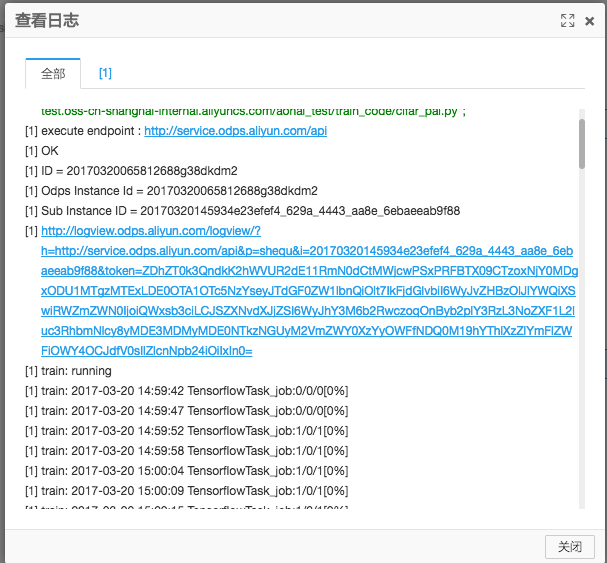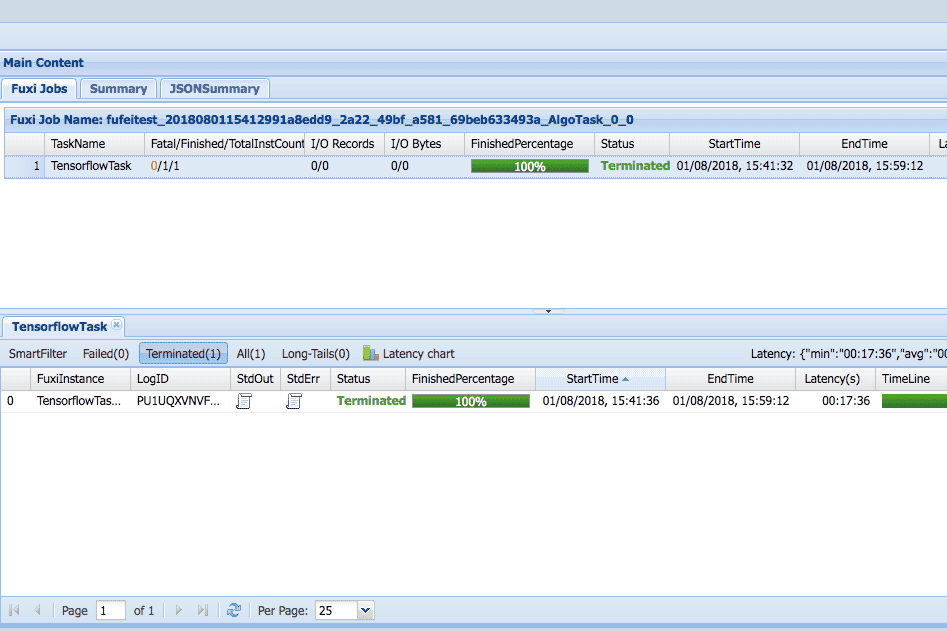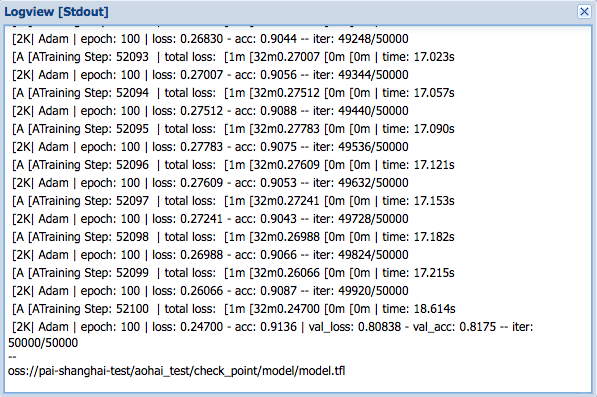### 5.结果预测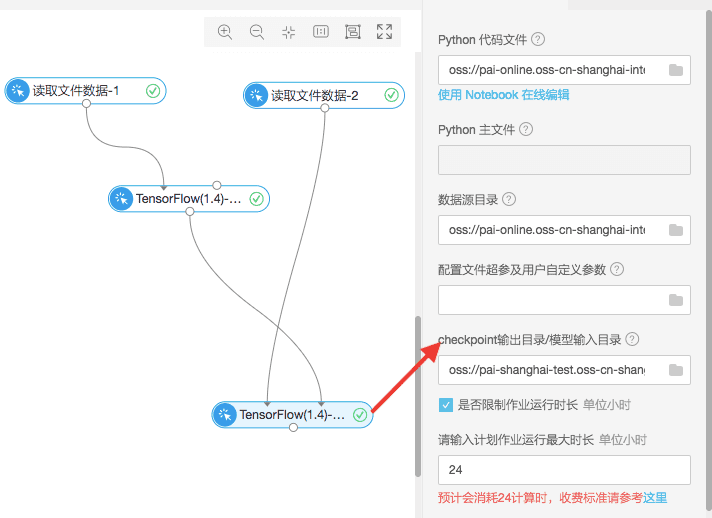• Python代码文件：OSS中的cifar_predict_pai.py
• 数据源目录：OSS中的cifar-10-batches-py文件夹,用来读取bird_mount_bluebird.jpg文件，从读文件数据组件自动同步
• checkpoint输出目录/模型输入目录：需要跟tensorflow训练组间的模型输出目录一致

#### 5.1预测代码数据

``  predict_pic = os.path.join(FLAGS.buckets, "bird_bullocks_oriole.jpg")``    img_obj = file_io.read_file_to_string(predict_pic)``    file_io.write_string_to_file("bird_bullocks_oriole.jpg", img_obj)``    img = scipy.ndimage.imread("bird_bullocks_oriole.jpg", mode="RGB")``    # Scale it to 32x32``    img = scipy.misc.imresize(img, (32, 32), interp="bicubic").astype(np.float32, casting='unsafe')``    # Predict``    prediction = model.predict([img])``    print (prediction)``    print (prediction)``    #print (prediction.index(max(prediction)))``    num=['airplane','automobile','bird','cat','deer','dog','frog','horse','ship','truck']``    print ("This is a %s"%(num[prediction.index(max(prediction))]))``